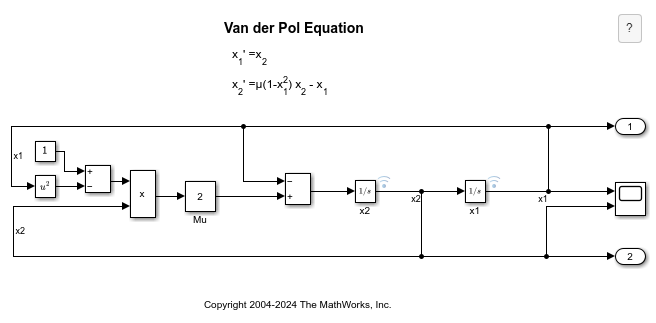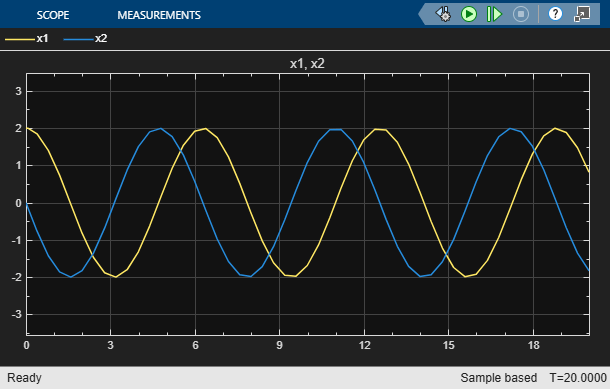# Van der Pol Oscillator

This example shows how to model the second-order Van der Pol (VDP) differential equation in Simulink®. In dynamics, the VDP oscillator is non-conservative and has nonlinear damping. At high amplitudes, the oscillator dissipates energy. At low amplitudes, the oscillator generates energy. The oscillator is given by this second-order differential equation:where:

• x is position as a function of time.

• Mu is damping.

The VDP oscillator is used in physical and biological sciences, including electric circuits.

open_system('vdp');### Simulate with Mu = 1

When Mu = 1, the VDP oscillator has nonlinear damping.

set_param('vdp/Mu','Gain','1') sim('vdp'); open_system('vdp/Scope');### Simulate with Mu = 0

When Mu = 0, the VDP oscillator has no damping. Energy is conserved in this simple harmonic oscillator. The equation becomes:set_param('vdp/Mu','Gain','0') sim('vdp'); open_system('vdp/Scope');